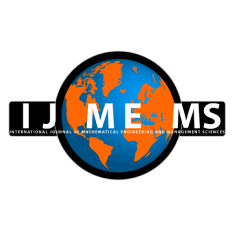### International Journal of Mathematical, Engineering and Management Sciences

#### ISSN: 2455-7749

An Approach for Solving Fuzzy Multi-Objective Linear Fractional Programming Problems

#### An Approach for Solving Fuzzy Multi-Objective Linear Fractional Programming Problems

Farhana Akond Pramy

;
Accepted on December 23, 2017

Abstract

In this paper, an attempt has been taken to develop a method for solving fuzzy multi-objective linear fractional programming (FMOLFP) problem. Here, at first the FMOLFP problem is converted into (crisp) multi-objective linear fractional programming (MOLFP) problem using the graded mean integration representation (GMIR) method proposed by Chen and Hsieh. That is, all the fuzzy parameters of FMOLFP problem are converted into crisp values. Then the MOLFP problem is transformed into a single objective linear programming (LP) problem using a proposal given by Nuran Guzel. Finally the single objective LP problem is solved by regular simplex method which yields an efficient solution of the original FMOLFP problem. To show the efficiency of our proposed method, three numerical examples are illustrated and also for each example, a comparison is drawn between our proposed method and the respected existing method.

Keywords- Fuzzy set, Linear fractional programming (LFP), Multi-objective linear fractional programming (MOLFP), Fuzzy multi-objective linear fractional programming (FMOLFP), Graded mean integration representation (GMIR).

Citation

Pramy, F. A (2018). An Approach for Solving Fuzzy Multi-Objective Linear Fractional Programming Problems. International Journal of Mathematical, Engineering and Management Sciences, 3(3), 280-293. https://dx.doi.org/10.33889/IJMEMS.2018.3.3-020.

Conflict of Interest

Acknowledgements

References

Bajalinov, E. B. (2003). Linear-fractional programming: theory, methods, applications and software. Kluwer Academic Publishers, Boston.

Bellman, R. E., & Zadeh, L.A. (1970). Decision making in a fuzzy environment. Management Science, 17, 141-164.

Benson, H. P. (1985). Finding certain weakly-efficient vertices in multiple objective linear fractional programming. Management Science, 31(2), 240-245.

Charnes, A., & Cooper, W. W. (1962). Programming with linear fractional functionals. Naval Research Logistics, 9(3-4), 181-186.

Chen, S. H., & Hsieh, C. H. (1999). Graded mean integration representation of generalized fuzzy number. Journal of Chinese Fuzzy Systems, 5(2), 1-7.

Dheyab, A. N. (2012). Finding the optimal solution for fractional linear programming problems with fuzzy numbers. Journal of Kerbala University, 10(3), 105-110

Dinkelbach, W. (1967). On nonlinear fractional programming. Management Science, 13(7), 492-498.

Guzel, N. (2013, November). A proposal to the solution of multiobjective linear fractional programming problem. Abstract and Applied Analysis (Vol. 2013). Hindawi Publishing Corporation.

Guzel, N., & Sivri, M. (2005). Proposal of a solution to multi objective linear fractional programming problem. Sigma Journal of Engineering and Natural Sciences, 2, 43–50.

Jain, S. (2012). Modeling of Gauss elimination technique for multi-objective linear programming problem. Journal of Novel Applied Sciences, 1, 25-29.

Jain, S. (2014). Modeling of Gauss elimination technique for multi-objective fractional programming problem. South Asian Journal of Mathematics, 4(3), 148-153.

Kornbluth, J. S., & Steuer, R. E. (1981). Multiple objective linear fractional programming. Management Science, 27(9), 1024-1039.

Moumita, D., & De, P. K. (2014). A note on fuzzy multi-objective linear fractional programming problem. International Journal of Computer Science and Network, 3(6), 568-572.

Muruganandam, S., & Ambika, P. (2017). Harmonic mean technique to solve multi objective fuzzy linear fractional programming problems. Global Journal of Pure and Applied Mathematics, 13(10), 7321-7329.

Porchelvi, R. S., Vasanthi L., & Hepzibah, R. I. (2014). On solving multi objective fractional linear programming problems. International Journal of Current Research, 6(8), 8095-8102.

Swarup, K. (1965). Linear fractional functionals programming. Operations Research, 13(6), 1029-1036.